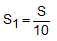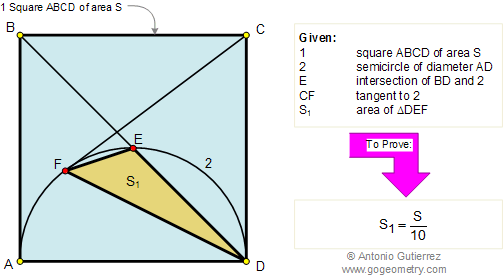Problem 112. Area of Square and Triangle. Level: High School, SAT Prep, College

 In the figure below, given an square ABCD of area S, E is the intersection of BD and the semicircle of diameter AD, and CF is tangent to the semicircle. If S1 is the area of triangle DEF, prove that:.## Geometric Art: Hyperbolic Kaleidoscope of problem 112 using iPad Apps: Equilateral Triangles and Squares.
 Home | Search | Problems | 111-120 | Circle Tangent Line | Circle Inscribed in a Square | Email | View or post a solution | by Antonio Gutierrez